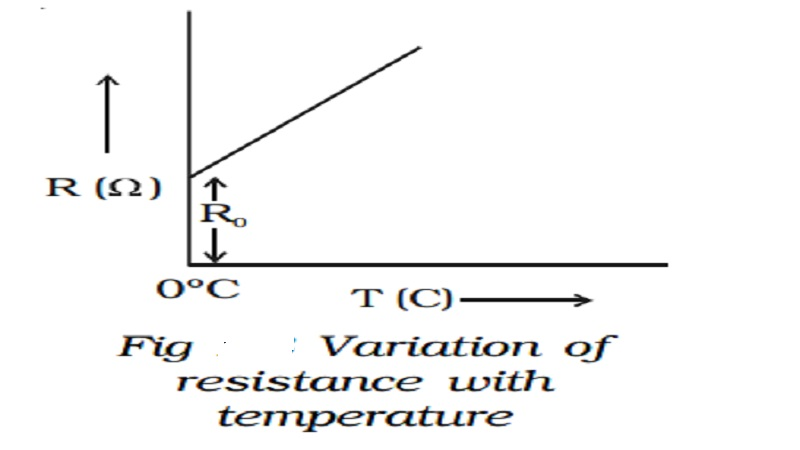Home | | Physics | | Physics | Temperature dependence of resistance and Internal resistance of a cell

# Temperature dependence of resistance and Internal resistance of a cellThe resistivity of substances varies with temperature. For conductors the resistance increases with increase in temperature.

## Temperature dependence of resistance

The resistivity of substances varies with temperature. For conductors the resistance increases with increase in temperature. If Ro is the resistance of a conductor at 0o C and Rt is the resistance of same conductor at to C, then

Rt = Ro (1 + αt)

where  α  is  called  the  temperature coefficient of resistance.The temperature coefficient of resistance is defined as the ratio of increase in resistance per degree rise in temperature to its resistance at 0o C. Its unit is per oC.

The variation of resistance with temperature is shown in diagram.

Metals have positive temperature coefficient of resistance, i.e., their resistance increases with increase in temperature. Insulators and semiconductors have negative temperature coefficient of resistance, i.e., their resistance decreases with increase in temperature. A material with a negative temperature coefficient is called a thermistor. The temperature coefficient is low for alloys.

## Internal resistance of a cell

The electric current in an external circuit flows from the positive terminal to the negative terminal of the cell, through different circuit elements. In order to maintain continuity, the current has to flow through the electrolyte of the cell, from its negative terminal to positive terminal. During this process of flow of current inside the cell, a resistance is offered to current flow by the electrolyte of the cell. This is termed as the internal resistance of the cell.

A freshly prepared cell has low internal resistance and this increases with ageing.

Determination of internal resistance of a cell using voltmeter

The circuit connections are made as shown in Fig 2.9. With key K open, the emf of cell E is found by connecting a high resistance voltmeter across it.  Since the high resistance voltmeter draws only a very feeble current for deflection, the circuit may be considered as an open circuit. Hence the voltmeter reading gives the emf of the cell. A small value of resistance R is included in the external circuit and key K is closed. The potential difference across R is equal to the potential difference across cell (V).The potential drop across R, V = IR         .. .. .. .. (1)

Due to internal resistance r of the cell, the voltmeter reads a value V, less than the emf of cell.

Then   V = E - Ir  or  Ir = E-V         ...(2)

Dividing equation (2) by equation (1)Since E, V and R are known, the internal resistance r of the cell can be determined.

Study Material, Lecturing Notes, Assignment, Reference, Wiki description explanation, brief detail

Related Topics